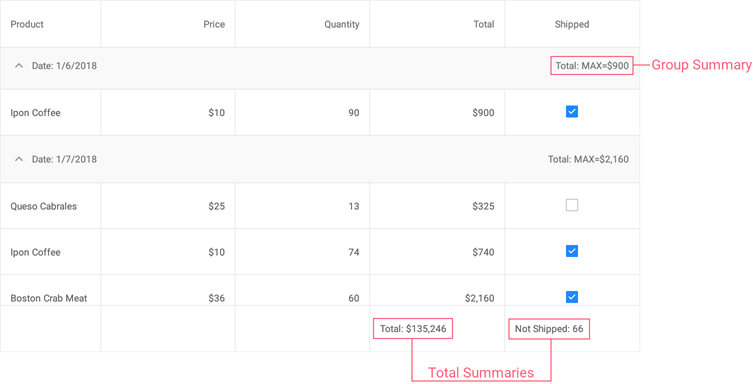# GridColumnSummary Class

A data summary item.

Namespace: DevExpress.Maui.DataGrid

Assembly: DevExpress.Maui.DataGrid.dll

## Declaration

``````public class GridColumnSummary :
BindableObject,
IXtraSerializableLayoutEx``````

## Remarks

DataGridView can automatically calculate summaries for individual data columns or groups of rows. For example, it can sum values, count the number of records, detect a minimum or maximum value, etc.

The grid supports two summary types: total summaries (calculated over all rows in a column and displayed below this column) and group summaries (calculated over all rows in a group and displayed in a group row).

To create a total or group summary, add a GridColumnSummary object to the DataGridView.TotalSummaries or DataGridView.GroupSummaries collection. Use the following properties to adjust summary settings:

## Example

This example uses predefined aggregate functions (Max and Sum) and a custom rule to calculate group and total summaries for a grid that displays orders grouped by dates.Set up the following summaries:

• A group summary to display the maximum Total value for each group of orders.
• A total summary to calculate the sum of values in the Total column.
• A custom total summary to count the number of orders with the false value in the Shipped column.
``````<dxg:DataGridView x:Name="grid" ItemsSource="{Binding Orders}"
CalculateCustomSummary="grid_CalculateCustomSummary">
<!-- ... -->
<dxg:DataGridView.GroupSummaries>
<dxg:GridColumnSummary FieldName="Total" Type="Max"/>
</dxg:DataGridView.GroupSummaries>

<dxg:DataGridView.TotalSummaries>
<dxg:GridColumnSummary FieldName="Total" Type="Sum"
DisplayFormat="Total: {0:C0}"/>
<dxg:GridColumnSummary FieldName="Shipped" Type="Custom"
DisplayFormat="Not Shipped: {0}"/>
</dxg:DataGridView.TotalSummaries>
</dxg:DataGridView>
``````
``````int count;
// ...

void grid_CalculateCustomSummary(object sender, DevExpress.Maui.DataGrid.CustomSummaryEventArgs e) {
if (e.FieldName.ToString () == "Shipped")
if (e.IsTotalSummary){
if (e.SummaryProcess == CustomSummaryProcess.Start) {
count = 0;
}
if (e.SummaryProcess == CustomSummaryProcess.Calculate) {
if (!(bool)e.FieldValue)
count++;
e.TotalValue = count;
}
}
}
``````

## Inheritance

Object
Microsoft.Maui.Controls.BindableObject
GridColumnSummary